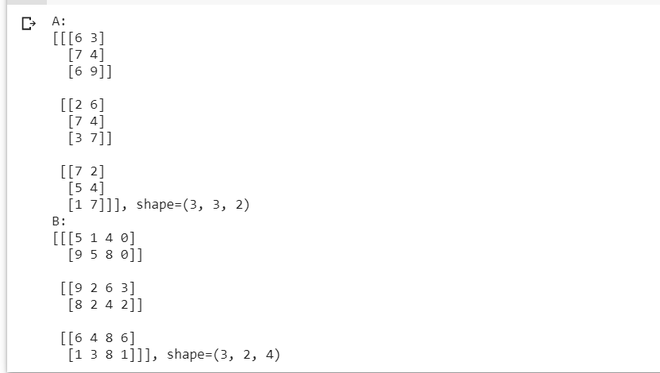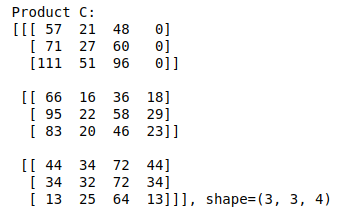# NumPy – 3D matrix multiplication

• Difficulty Level : Easy
• Last Updated : 12 Nov, 2020

A 3D matrix is nothing but a collection (or a stack) of many 2D matrices, just like how a 2D matrix is a collection/stack of many 1D vectors. So, matrix multiplication of 3D matrices involves multiple multiplications of 2D matrices, which eventually boils down to a dot product between their row/column vectors.

Let us consider an example matrix A of shape (3,3,2) multiplied with another 3D matrix B of shape (3,2,4).

Attention geek! Strengthen your foundations with the Python Programming Foundation Course and learn the basics.

To begin with, your interview preparations Enhance your Data Structures concepts with the Python DS Course. And to begin with your Machine Learning Journey, join the Machine Learning - Basic Level Course

## Python

 `import` `numpy as np`` ` `np.random.seed(``42``)`` ` `A ``=` `np.random.randint(``0``, ``10``, size``=``(``3``, ``3``, ``2``))``B ``=` `np.random.randint(``0``, ``10``, size``=``(``3``, ``2``, ``4``))`` ` `print``(``"A:\n{}, shape={}\nB:\n{}, shape={}"``.``format``(``  ``A, A.shape, B, B.shape))`

OUTPUT:The first matrix is a stack of three 2D matrices each of shape (3,2), and the second matrix is a stack of 3 2D matrices, each of shape (2,4).

The matrix multiplication between these two will involve three multiplications between corresponding 2D matrices of A and B having shapes (3,2) and (2,4) respectively. Specifically, the first multiplication will be between A and B, the second multiplication will be between A and B, and finally, the third multiplication will be between A and B. The result of each individual multiplication of 2D matrices will be of shape (3,4). Hence, the final product of the two 3D matrices will be a matrix of shape (3,3,4).

Let’s realize this using code.

## Python

 `C ``=` `np.matmul(A, B)`` ` `print``(``"Product C:\n{}, shape={}"``.``format``(C, C.shape))`

Output:My Personal Notes arrow_drop_up